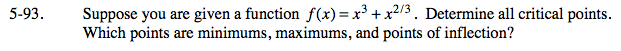### Home > CALC > Chapter 5 > Lesson 5.2.4 > Problem5-93

5-93.

Suppose you are given a function f(x) = x3 + x2/3. Determine all critical points. Which points are minimums, maximums, and points of inflection? Homework Help ✎Find the critical points by setting both f '(x) and f "(x) equal to zero and by finding where f '(x) does not exist.